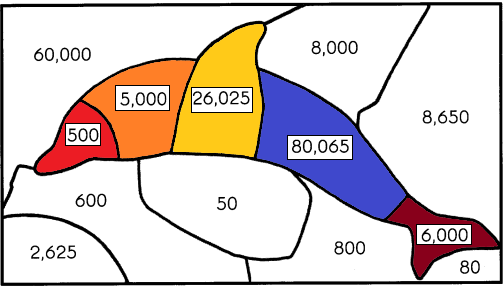# Math in Focus Grade 4 Chapter 1 Practice 2 Answer Key Numbers to 10,000

Go through the Math in Focus Grade 4 Workbook Answer Key Chapter 1 Practice 2 Numbers to 10,000 to finish your assignments.

## Math in Focus Grade 4 Chapter 1 Practice 2 Answer Key Numbers to 10,000

Complete.

In 71,486,
Example
the digit 7 is in the thousands place.

Question 1.
the digit 1 is in the ____ place.
The above-given number is 71,486
In that number, we need to find out the place value of digit 1.
Definition of place value: In Mathematics, place value charts help us to make sure that the digits are in the correct places. To identify the positional values of numbers accurately, first, write the digits in the place value chart and then write the numbers in the usual and the standard form.
– A number system is a method of representing numbers. It is also known as the enumeration scheme, which determines a set of values to describe a quantity. The position of a digit in a number determines the value of the digit in it. For instance, 5 in 350 represents 5 tens, or 50; but 5 in 5,006 represents 5,000. Kids need to know that while the same digit can be present in many numbers, its value depends on where it is in the number.
– According to the above definition, in the number 71,486:
– 1 holds the thousands position.

Question 2.
the digit 4 is in the ____ place.
Explanation:
The above-given number is 71,486
In that number, we need to find out the place value of digit 4.
Definition of place value: In Mathematics, place value charts help us to make sure that the digits are in the correct places. To identify the positional values of numbers accurately, first, write the digits in the place value chart and then write the numbers in the usual and the standard form.
– A number system is a method of representing numbers. It is also known as the enumeration scheme, which determines a set of values to describe a quantity. The position of a digit in a number determines the value of the digit in it. For instance, 5 in 350 represents 5 tens, or 50; but 5 in 5,006 represents 5,000. Kids need to know that while the same digit can be present in many numbers, its value depends on where it is in the number.
– According to the above definition, in the number 71,486:
– 4 holds the hundreds position.

Question 3.
the digit 8 is in the ____ place.
Explanation:
The above-given number is 71,486
In that number, we need to find out the place value of the digit 8.
Definition of place value: In Mathematics, place value charts help us to make sure that the digits are in the correct places. To identify the positional values of numbers accurately, first, write the digits in the place value chart and then write the numbers in the usual and the standard form.
– A number system is a method of representing numbers. It is also known as the enumeration scheme, which determines a set of values to describe a quantity. The position of a digit in a number determines the value of the digit in it. For instance, 5 in 350 represents 5 tens, or 50; but 5 in 5,006 represents 5,000. Kids need to know that while the same digit can be present in many numbers, its value depends on where it is in the number.
– According to the above definition, in the number 71,486:
– 8 holds the tens position.

Question 4.
the digit 6 is in the ____ place.
Explanation:
The above-given number is 71,486
In that number, we need to find out the place value of the digit 6.
Definition of place value: In Mathematics, place value charts help us to make sure that the digits are in the correct places. To identify the positional values of numbers accurately, first, write the digits in the place value chart and then write the numbers in the usual and the standard form.
– A number system is a method of representing numbers. It is also known as the enumeration scheme, which determines a set of values to describe a quantity. The position of a digit in a number determines the value of the digit in it. For instance, 5 in 350 represents 5 tens, or 50; but 5 in 5,006 represents 5,000. Kids need to know that while the same digit can be present in many numbers, its value depends on where it is in the number.
– According to the above definition, in the number 71,486:
– 6 holds the one’s position.

Find the value of each digit.

In 65,239,

Example
the digit 6 stands for 60,000

Question 5.
the digit 5 stands for _________
Explanation:
The above-given number is 65,239
We need to write a particular place value.
– 5 is in the thousands place.
In number system, we can write as:5000
In Mathematics, place value charts help us to make sure that the digits are in the correct places. To identify the positional values of numbers accurately, first, write the digits in the place value chart and then write the numbers in the usual and the standard form.

Question 6.
the digit 2 stands for _________
Explanation:
The above-given number is 65,239
We need to write a particular place value.
– 2 is in the hundreds place.
In number system, we can write as:200
In Mathematics, place value charts help us to make sure that the digits are in the correct places. To identify the positional values of numbers accurately, first, write the digits in the place value chart and then write the numbers in the usual and the standard form.

Question 7.
the digit 3 stands for _________
Explanation:
The above-given number is 65,239
We need to write a particular place value.
– 3 is in the tens place.
In number system, we can write as:30
In Mathematics, place value charts help us to make sure that the digits are in the correct places. To identify the positional values of numbers accurately, first, write the digits in the place value chart and then write the numbers in the usual and the standard form.

Question 8.
the digit 9 stands for _________
Explanation:
The above-given number is 65,239
We need to write a particular place value.
– 9 is in the one’s place.
In number system, we can write as:9
In Mathematics, place value charts help us to make sure that the digits are in the correct places. To identify the positional values of numbers accurately, first, write the digits in the place value chart and then write the numbers in the usual and the standard form.

Write each number using the clues.

Question 9.
The value of digit 1 is 100.
The value of digit 5 is 50.
The value of digit 3 is 3.
The value of digit 4 is 40,000.
The value of digit 2 is 2,000.

The number is ____
Explanation:
In Mathematics, place value charts help us to make sure that the digits are in the correct places. To identify the positional values of numbers accurately, first, write the digits in the place value chart and then write the numbers in the usual and the standard form.
The above-given clues are given in a form of numbers. By using the place values we can write the number.
The format of the place value chart: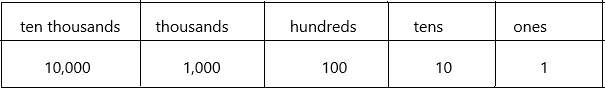According to the chart write the number by using clues:
– First, see the highest number and check its place value.
– The highest number is 40,000. It should be in the ten thousand position.
– The next least number is 2,000. It should be in the thousands position.
– The next least number is 100. It should be in the hundreds position.
– The next least number is 50. It should be in the tens position.
– The next least number is 3. It should be in the one’s position.
– Therefore, the overall number format is 42,153.

Question 10.
The digit 4 is in the hundreds place.
The digit 2 is in the ten thousands place.
The digit 9 is in the tens place.
The digit 0 is in the ones place.

The number is ____
Explanation:
In Mathematics, place value charts help us to make sure that the digits are in the correct places. To identify the positional values of numbers accurately, first, write the digits in the place value chart and then write the numbers in the usual and the standard form.
The above-given clues are given in a form of numbers. By using the place values we can write the number.
The format of the place value chart:According to the chart write the number by using clues:
– First, see the highest number and check its place value.
– The highest number is 20,000. It should be in the ten thousand position.
– There is no thousands place. So we should keep ‘0’ in the thousands position.
– The next least number is 400. It should be in the hundreds position.
– The next least number is 90. It should be in the tens position.
– The next least number is 0. It should be in the one’s position.
– Therefore, the overall number format is 20,490.

Write the missing numbers and words.

Example

In 36,172,
the digit 2 stands for 2 ones.
the digit 6 is in thousands place.
the digit in the ten thousands place is 3
the value of the digit 7 is 70
the digit 1 is in the hundreds place an its value is 100.

write the missing numbers and words.

In 52,814,

Question 11.
the digit 4 stands for ______________ ones.
Place value in Maths describes the position or place of a digit in a number. Each digit has a place in a number. When we represent the number in general form, the position of each digit will be expanded. Those positions start from a unit place or we also call it one’s position. The order of place value of digits of a number of right to left is units, tens, hundreds, thousands, ten thousand, a hundred thousand, and so on.
The place value chart:According to the chart write the place value of 4:
The above-given number is 52,814
– A number in one’s place is 4
– 4 one’s is nothing but 4

Question 12.
the digit 1 is in the ___ place.
Place value in Maths describes the position or place of a digit in a number. Each digit has a place in a number. When we represent the number in general form, the position of each digit will be expanded. Those positions start from a unit place or we also call it one’s position. The order of place value of digits of a number of right to left is units, tens, hundreds, thousands, ten thousand, a hundred thousand, and so on.
The place value chart:According to the chart write the place value of 1:
The above-given number is 52,814
– A number in tens place is 1
– 1 ten is nothing but 10.

Question 13.
the digit in the ten thousands place is ____
Place value in Maths describes the position or place of a digit in a number. Each digit has a place in a number. When we represent the number in general form, the position of each digit will be expanded. Those positions start from a unit place or we also call it one’s position. The order of place value of digits of a number of right to left is units, tens, hundreds, thousands, ten thousand, a hundred thousand, and so on.
The place value chart:According to the chart write the ten thousands place:
The above-given number is 52,814
– A number in ten thousands place is 5
– 5 ten thousand is nothing but 50,000

Question 14.
the value of the digit 8 is ____.
Place value in Maths describes the position or place of a digit in a number. Each digit has a place in a number. When we represent the number in general form, the position of each digit will be expanded. Those positions start from a unit place or we also call it one’s position. The order of place value of digits of a number of right to left is units, tens, hundreds, thousands, ten thousand, a hundred thousand, and so on.
The place value chart:According to the chart write the place value of 8:
The above-given number is 52,814
– A number in the hundreds place is 8
– 8 hundred is nothing but 800.

Question 15.
the digit ___ is in the thousands place and its value is ____.
Answer: 2 is in the thousands place and its value is 2000
Explanation:
Place value in Maths describes the position or place of a digit in a number. Each digit has a place in a number. When we represent the number in general form, the position of each digit will be expanded. Those positions start from a unit place or we also call it one’s position. The order of place value of digits of a number of right to left is units, tens, hundreds, thousands, ten thousand, a hundred thousand, and so on.
The place value chart:According to the chart write the thousands place:
The above-given number is 52,814
– A number in the thousands place is 2
– 2 thousand is nothing but 2000.

Complete.

Example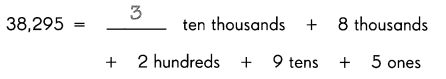Question 16.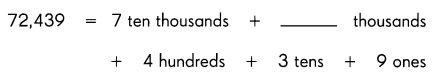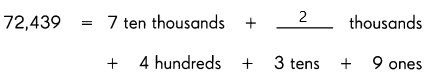Explanation:
The standard form is given:72,439
Now we can write in expanded form: The expanded form of the numbers helps to determine the place value of each digit in the given number. It means that the expansion of numbers is based on the place value. The expanded form splits the number, and it represents the number in units, tens, hundreds and thousands form.
7 is in the ten thousand positions.
2 is in the thousands position
4 is in the hundreds position.
3 is in the tens position.
9 is in the one’s position
These numbers we can write according to their place values with its position values.
Therefore, the expanded form:
70,000+2,000+400+30+9.

Question 17.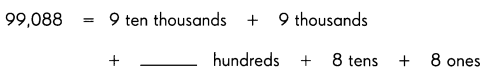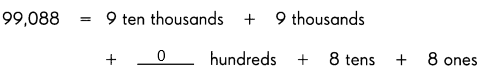Explanation:
The standard form is given:99,088
Now we can write in expanded form: The expanded form of the numbers helps to determine the place value of each digit in the given number. It means that the expansion of numbers is based on the place value. The expanded form splits the number, and it represents the number in units, tens, hundreds and thousands form.
9 is in the ten thousand positions.
9 is in the thousands position
0 is in the hundreds position.
2 is in the tens position.
8 is in the one’s position
These numbers we can write according to their place values with its position values.
Therefore, the expanded form:
90,000+9,000+20+8.

Complete the expanded form.

Example
51,476 = 50,000 + 1,000 + 400 + 70 + 6

Question 18.
36,427 = 30,000 + _________ + 400 + 20 + 7
The expanded form of the numbers helps to determine the place value of each digit in the given number. It means that the expansion of numbers is based on the place value. The expanded form splits the number, and it represents the number in units, tens, hundreds and thousands form.
How to write expanded form:
Go through the below steps to write the numbers in expanded form:
Step 1: Get the standard form of the number.
Step 2Identify the place value of the given number using the place value chart.
Step 3: Multiply the given digit by its place value and represent the number in the form of (digit × place value).
Step 4: Finally, represent all the numbers as the sum of (digit × place value) form, which is the expanded form of the number.
Now write according to the above steps:
1. The standard form of a number is 36,427
2. The place value of the given number is:
3 is in the ten thousand positions.
6 is in the thousands position
4 is in the hundreds position.
2 is in the tens position.
7 is in the one’s position.
3. Multiply the given number by its place value.
(i.e.,) 3×10, 000, 6×1000, 4×100, 2×10, 7×1
4. Expanded form is 30,000+ 6000+400+20+7
Finally, the expanded form of the number 36,427 is 30,000+ 6000+400+20+7.

Question 19.
17,503 = 10,000 + 7,000 + ________ + 3
The expanded form of the numbers helps to determine the place value of each digit in the given number. It means that the expansion of numbers is based on the place value. The expanded form splits the number, and it represents the number in units, tens, hundreds and thousands form.
How to write expanded form:
Go through the below steps to write the numbers in expanded form:
Step 1: Get the standard form of the number.
Step 2Identify the place value of the given number using the place value chart.
Step 3: Multiply the given digit by its place value and represent the number in the form of (digit × place value).
Step 4: Finally, represent all the numbers as the sum of (digit × place value) form, which is the expanded form of the number.
Now write according to the above steps:
1. The standard form of a number is 17,503
2. The place value of the given number is:
– 1 is in the ten thousand positions.
– 7 is in the thousands position
– 5 is in the hundreds position.
– 0 is in the tens position.
– 3 is in the one’s position.
3. Multiply the given number by its place value.
(i.e.,) 1×10, 000, 7×1000, 5×100, 0×10, 3×1
4. Expanded form is 10,000+ 7000+500+0+3
Finally, the expanded form of the number 17,503 is 10,000+ 7000+500+0+3.

Question 20.
45,080 = 40,000 + _________ + 80
The expanded form of the numbers helps to determine the place value of each digit in the given number. It means that the expansion of numbers is based on the place value. The expanded form splits the number, and it represents the number in units, tens, hundreds and thousands form.
How to write expanded form:
Go through the below steps to write the numbers in expanded form:
Step 1: Get the standard form of the number.
Step 2Identify the place value of the given number using the place value chart.
Step 3: Multiply the given digit by its place value and represent the number in the form of (digit × place value).
Step 4: Finally, represent all the numbers as the sum of (digit × place value) form, which is the expanded form of the number.
Now write according to the above steps:
1. The standard form of a number is 45,080
2. The place value of the given number is:
– 4 is in the ten thousand positions.
– 5 is in the thousands position
– 0 is in the hundreds position.
– 8 is in the tens position.
– 0 is in the one’s position.
3. Multiply the given number by its place value.
(i.e.,) 4×10, 000, 5×1000, 0×100, 8×10, 0×1
4. Expanded form is 40,000+ 5000+0+80+0
Finally, the expanded form of the number 45,080 is 40,000+ 5000+0+80+0.

Question 21.
20,000 + 6,000 + 20 + 5 = _________
– A standard form of a number in Maths is basically mentioned for the representation of large numbers or small numbers. We use exponents to represent such numbers in standard form.
– The correct definition of standard form could be explained better in terms of decimal numbers and following certain rules.  As we know, decimal numbers are the simplified form of fractions.
How to write the standard form of a number:
The steps to write the standard form of a number are as follows:
Step 1: Write the first number from the given number.
Step 2: Add the decimal point after the first number.
Step 3: Now, count the number of digits after the first number from the given number and write it in the power of  The given expanded form is 20,000 + 6,000 + 20 + 5. Thus the standard form of a number is obtained as follows:
Step 1: The first number is 2
Step 2: Adding the decimal point after 2, it becomes “2.”
Step 3: The number of digits after 2 is 4.
Hence, the standard form is 2.6× 104.
we can write in the number form also: 26,025

Question 22.
5 + 60 + 80,000 = ________
– A standard form of a number in Maths is basically mentioned for the representation of large numbers or small numbers. We use exponents to represent such numbers in standard form.
– The correct definition of standard form could be explained better in terms of decimal numbers and following certain rules.  As we know, decimal numbers are the simplified form of fractions.
How to write the standard form of a number:
The steps to write the standard form of a number are as follows:
Step 1: Write the first number from the given number.
Step 2: Add the decimal point after the first number.
Step 3: Now, count the number of digits after the first number from the given number and write it in the power of  The given expanded form is 5 + 60 + 80,000. Thus the standard form of a number is obtained as follows:
Step 1: The first number is 8
Step 2: Adding the decimal point after 8, it becomes “8.”
Step 3: The number of digits after 8 is 4.
Hence, the standard form is 8.0× 104.
we can write in the number form also: 80,065.

Solve.

Question 23.
Color the puzzle pieces that show the answers in Exercises 18 to 22.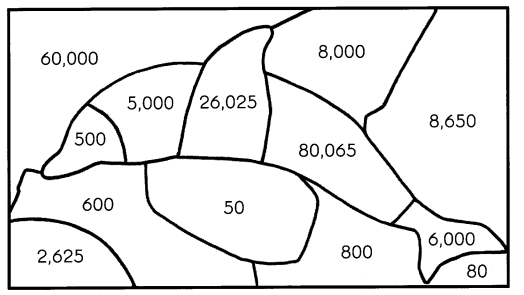What is this picture?
_________